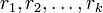# Criterion for element of alternating group to be real

## Statement

Suppose$n$ is a natural number and$A_n$ is the Alternating group (?) on a set of size$n$. An element$g \in A_n$ is a Real element (?) if and only if the cycle decomposition of$g$ satisfies one of these three conditions:

1. The cycle decomposition has a cycle of even length.
2. The cycle decomposition has two cycles of equal odd length. Note that fixed points are counted as cycles of length 1, so this includes any permutation that has two or more fixed points.
3. All the cycles have distinct odd lengths$r_1,r_2,\dots,r_k$ and$\sum_{i=1}^k (r_i - 1)/2$ is even. In other words, the number of$r_i$s that are congruent to$3$ modulo$4$ is even.

Note that the permutations which satisfy condition (1) or (2) are precisely those whose conjugacy class is unsplit from the symmetric group, where every element is real. (see splitting criterion for conjugacy classes in the alternating group). (3) is the case of a conjugacy class that splits in$A_n$ but each element still remains with its inverse.